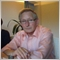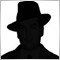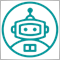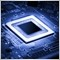• 概述
• 评论 (5)
• 评论
• 新特性

The Commodity Channel Index computes the deviation of the typical price of each bar from the average price over a particular time period. Multiplying the median deviation by 0.015 facilitates normalization, which makes all deviations below the first standard deviation less than  -100, and all deviations above the first standard deviation more than 100.

This adaptive version is based on the indicator described by John Ehlers, in the book Rocket Science for traders. The indicator uses the homodyne descriminator to compute the dominant cycle.

The cycle is calculated by taking the arctangent of the imaginary part divided by the real part. Restrictions are placed so as to make sure that the rate of change of the cycle period is limited to +-50% of the previous cycle period and further more, the resulting cycle period is limited to being greater than 6 and less than 50. Smoothing is then applied for the last time on the cycle period.

This cycle period value is then used at each bar close as the value that represents the number of bars back to be analyzed for producing the Commodity Channel Index. This enables the adaptive nature of the indicator.

It  can be used as an alternative to the conventional Commodity Channel Index.

Indicator Parameters:

• The limits value controls the number bars the indicator will be drawn for .
• The cyclepart is a modifier for the calculated cycle period, it helps to make sure that the indicator remains in phase with the price data. The figure can also be used to optimize the indicator for the measured cycle.1088
2020.06.14 17:56

Gute Arbeit, danke für diesen Indikator.1088
2020.06.14 17:56

Gute Arbeit, danke für diesen Indikator.[删除] 2019.01.14 07:2612010
2018.01.06 20:405715
2017.11.09 14:447196
2016.03.11 04:41

New version of the indicator has been optimized for the new MT5 environment.